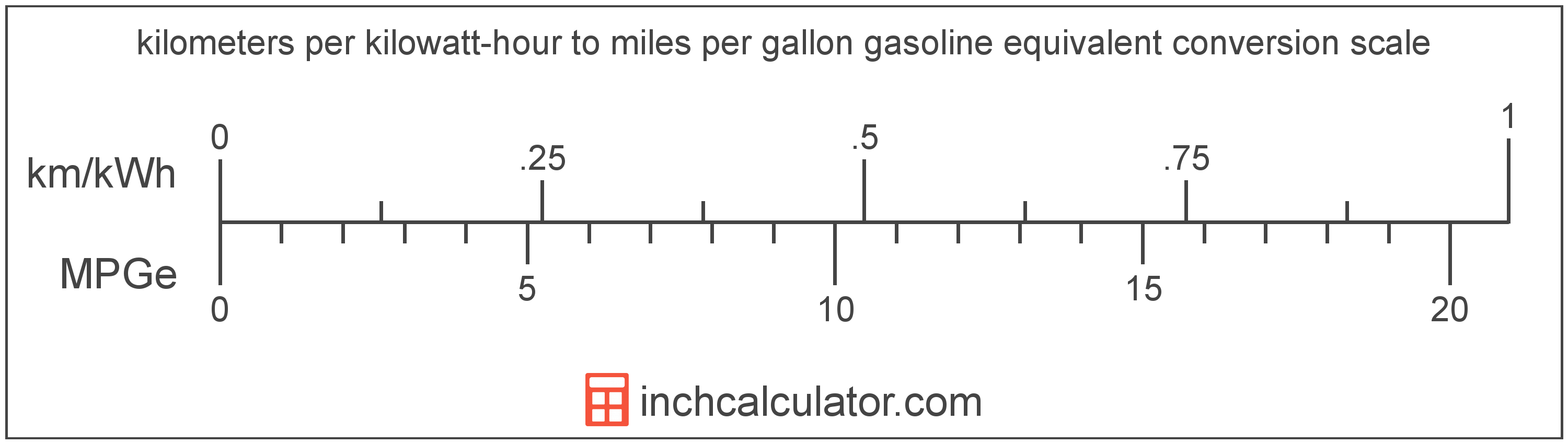# Kilometers per Kilowatt-Hour to Miles per Gallon Gasoline Equivalent Converter (Km/kWh to MPGe)

Enter the electric car efficiency in kilometers per kilowatt-hour below to get the value converted to miles per gallon gasoline equivalent.

Results in Miles per Gallon Gasoline Equivalent:1 km/kWh = 20.943316 MPGe

Do you want to convert MPGe to km/kWh?

## How to Convert Kilometers per Kilowatt-Hour to Miles per Gallon Gasoline Equivalent

Convert kilometers per kilowatt-hour to miles per gallon gasoline equivalent with this simple formula:

MPGe = km/kWh × 3370.5 ÷ 160.9344

Insert the km/kWh efficiency measurement in the formula and then solve to find the result.

For example, let's convert 50 km/kWh to MPGe:

50 km/kWh = ( 50 × 3370.5 ÷ 160.9344 ) = 1,047.165802 MPGeKilometers per kilowatt-hour and miles per gallon gasoline equivalent are both units used to measure electric car efficiency. Keep reading to learn more about each unit of measure.

## What Are Kilometers per Kilowatt-Hour?

Kilometers per kilowatt-hour is a measure of electric vehicle energy efficiency equivalent to the number of kilometers a vehicle can travel using one kilowatt-hour of energy.

The kilometer per kilowatt-hour is an SI unit of electric car efficiency in the metric system. Kilometers per kilowatt-hour can be abbreviated as km/kWh; for example, 1 kilometer per kilowatt-hour can be written as 1 km/kWh.

In the expressions of units, the slash, or solidus (/), is used to express a change in one or more units relative to a change in one or more other units.

## What Are Miles per Gallon Gasoline Equivalent?

Miles per gallon gasoline equivalent is a way of measuring the energy needed equivalent to a gallon of gasoline to travel a distance in miles.

Because electric vehicles do not consume gasoline, the EPA set an equivalent amount of energy in kilowatt-hours that is equal to one gallon of gasoline. According to the EPA, one gallon of gasoline is equal to 33.705 kilowatt-hours.

The mile per gallon gasoline equivalent is a US customary unit of electric car efficiency. Miles per gallon gasoline equivalent can be abbreviated as MPGe; for example, 1 mile per gallon gasoline equivalent can be written as 1 MPGe.

## Kilometer per Kilowatt-Hour to Mile per Gallon Gasoline Equivalent Conversion Table

Table showing various kilometer per kilowatt-hour measurements converted to miles per gallon gasoline equivalent.
Kilometers Per Kilowatt-hour Miles Per Gallon Gasoline Equivalent
1 km/kWh 20.94 MPGe
2 km/kWh 41.89 MPGe
3 km/kWh 62.83 MPGe
4 km/kWh 83.77 MPGe
5 km/kWh 104.72 MPGe
6 km/kWh 125.66 MPGe
7 km/kWh 146.6 MPGe
8 km/kWh 167.55 MPGe
9 km/kWh 188.49 MPGe
10 km/kWh 209.43 MPGe
11 km/kWh 230.38 MPGe
12 km/kWh 251.32 MPGe
13 km/kWh 272.26 MPGe
14 km/kWh 293.21 MPGe
15 km/kWh 314.15 MPGe
16 km/kWh 335.09 MPGe
17 km/kWh 356.04 MPGe
18 km/kWh 376.98 MPGe
19 km/kWh 397.92 MPGe
20 km/kWh 418.87 MPGe
21 km/kWh 439.81 MPGe
22 km/kWh 460.75 MPGe
23 km/kWh 481.7 MPGe
24 km/kWh 502.64 MPGe
25 km/kWh 523.58 MPGe
26 km/kWh 544.53 MPGe
27 km/kWh 565.47 MPGe
28 km/kWh 586.41 MPGe
29 km/kWh 607.36 MPGe
30 km/kWh 628.3 MPGe
31 km/kWh 649.24 MPGe
32 km/kWh 670.19 MPGe
33 km/kWh 691.13 MPGe
34 km/kWh 712.07 MPGe
35 km/kWh 733.02 MPGe
36 km/kWh 753.96 MPGe
37 km/kWh 774.9 MPGe
38 km/kWh 795.85 MPGe
39 km/kWh 816.79 MPGe
40 km/kWh 837.73 MPGe

## References

1. United States Environmental Protection Agency, Technology, https://www3.epa.gov/otaq/gvg/learn-more-technology.htm